# Verdvana

21 Nov 2019

### 1 AMD

#### 1.1 if A=4’b0011，B=3’b110 and C=4’b1110，then which one is the correct result for expression of {2{~^A}}^(B[1:0]&C[3:2]) ？

A. 00
B. 01
C. 10
D. 11

~^A = ~(0&0&1&1) = ~0 = 1;
{2{~^A}} = {2{1}} = 2’b11;
(B[1:0]&C[3:2]) = 2’b10 & 2’b11 = 2’b10;
{2{~^A}}^(B[1:0]&C[3:2]) = 2’b11 ^ 2’b10 = 2’b01.

#### 1.2 Which statement is correct？

A. UVM test termination is using raise/drop uvm_object.
B. When we set the verbosity to UVM_LOW,the message with UVM_NONE cannot be issued.
C. If we set the configuration object in test layer,both subcomponents and sequences can retrieve its handle.
D. In SV language,It fails to cast a null pointer to an object. “\$(abc,null);”

#### 1.12 Which type of timing path is NOT included in this picture?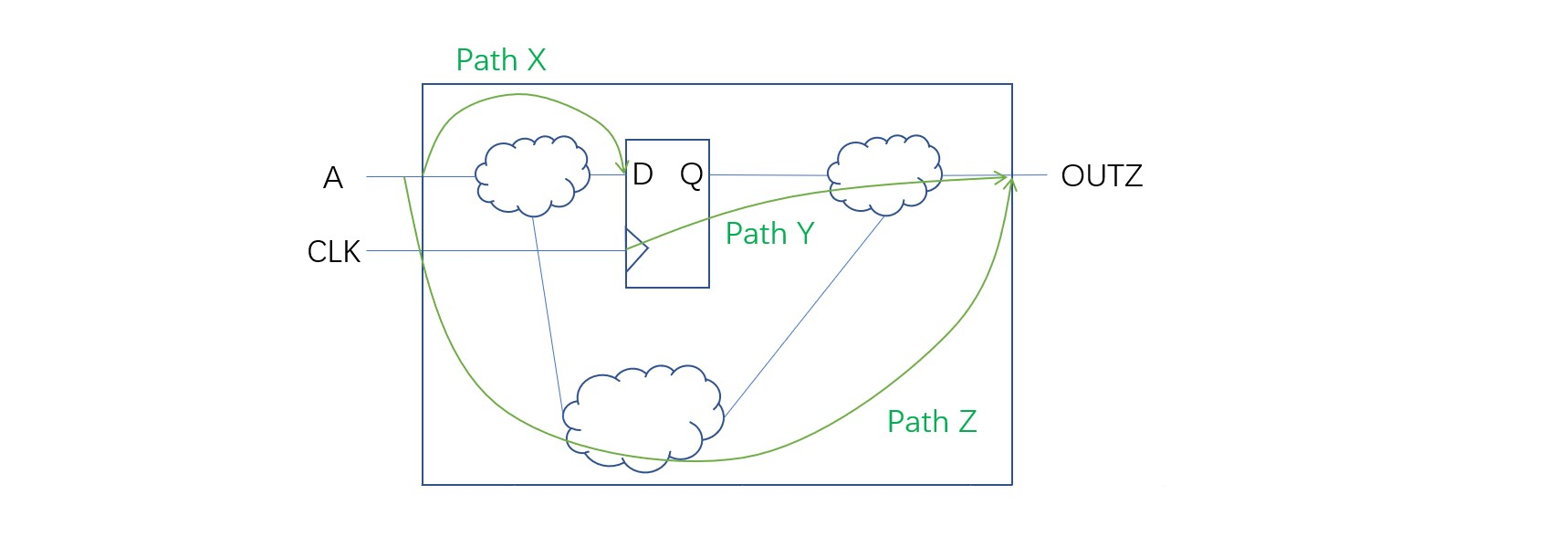A. Input port to a D pin of Flop.
B. CLK pin of One Flop to D pin next Flop.
C. Q pin of flop to an output port.
D. Input to output port through purely combinational logic.

#### 1.13 Which is the correct flow for Physical Design ?

A. Floorplan → Placement → CTS → Routing
B. CTS → Floorplan → Placement → Routing
C. Placement → Floorplan → CTS → Routing
D. CTS → Placement → Floorplan → Routing

The main steps in ASIC Physical design flow are:

• Design Netlist (after Synthesis);
• Floorplanning;
• Partitioning;
• Placement;
• Clock-Tree Synthesis (CTS);
• Routing;
• Physical Verification;
• GDS Ⅱ Generation。

A. Time.
B. Temperature.
C. Thickness.
D. Threshold.

A. Metal2.
B. Metal3.
C. Metal5.
D. Metal8.

A. SPEF.
B. DEF.
C. SDC.
D. Netlist.

STA tool:

• Netlist;
• Library;
• SDC;
• Parasitic.

#### 1.17 Which among the following methods are applicable to fix setup violation?

A. Swap high Vt cells into low Vt cells.
B. Increase driver size of long net.
C. Delaying the clock to the end point.
D. Decreasing the size of certain cells in the data path.

A. CK → D.
B. CK → Q.
C. D → Q.
D. CK → CDN.

#### 1.19 Cell Delay can be calculated based on:()

A. input transition.
C. output transition.

youwenti

#### 1.21 7nm工艺中的7nm指的是（）

A. 芯片中最小晶体管的源极长度.
B. 芯片中最小晶体管的源极宽度.
C. 芯片中最小单元的沟道长度.
D. 芯片中最小单元的源极宽度.

#### 1.22 What is the layer that TCP worked on？

A. Network.
C. Transport.
D. Application. E. Session.

OSI七层模型：

• 应用层；
• 表示层；
• 会话层；
• 传输层；
• 网络层；
• 数据链路层；
• 物理层。
TCP属于传输层；IP属于网络层；HTTP属于应用层，基于TCP连接。

A. 8.125V.
B. 4V.
C. 6.25V.
D. 9.375V.

#### 1.24 Which of below pattern can detect the fault in below diagram?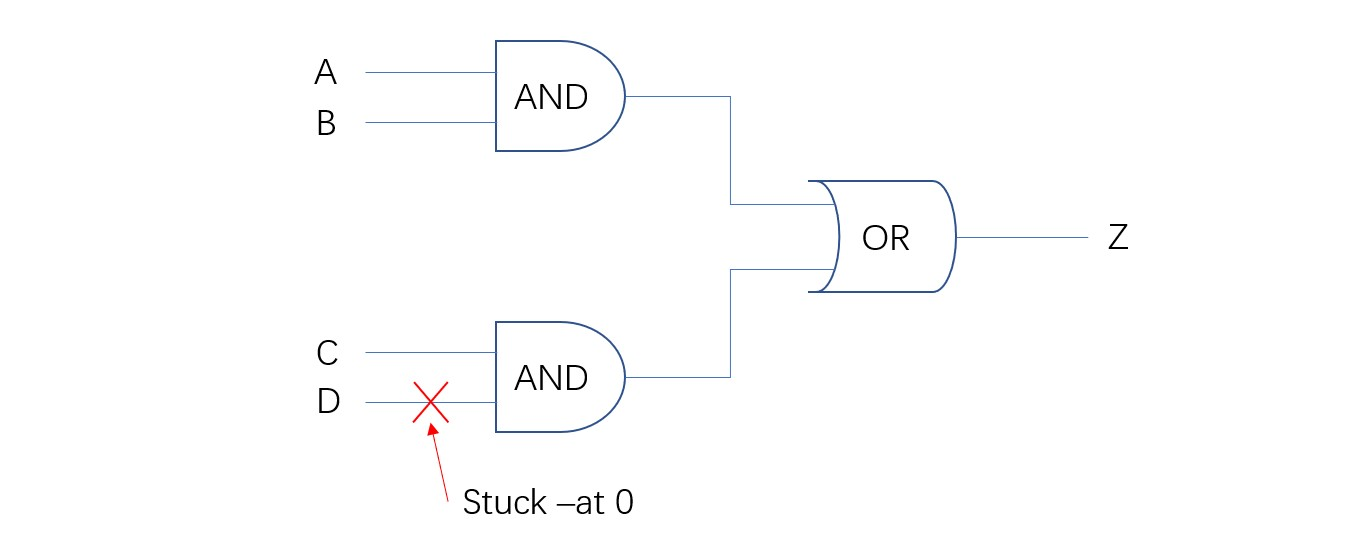A. A=1,B=1,C=0,D=0.
B. A=1,B=1,C=1,D=1.
C. A=0,B=0,C=1,D=1.
D. A=0,B=1,C=0,D=1.

#### 1.25 In ARM AMBA AHB protocol,which of following responses is ONE cycle response?

A. OKAY.
B. ERROR.
C. RETRY.
D. SPLIT.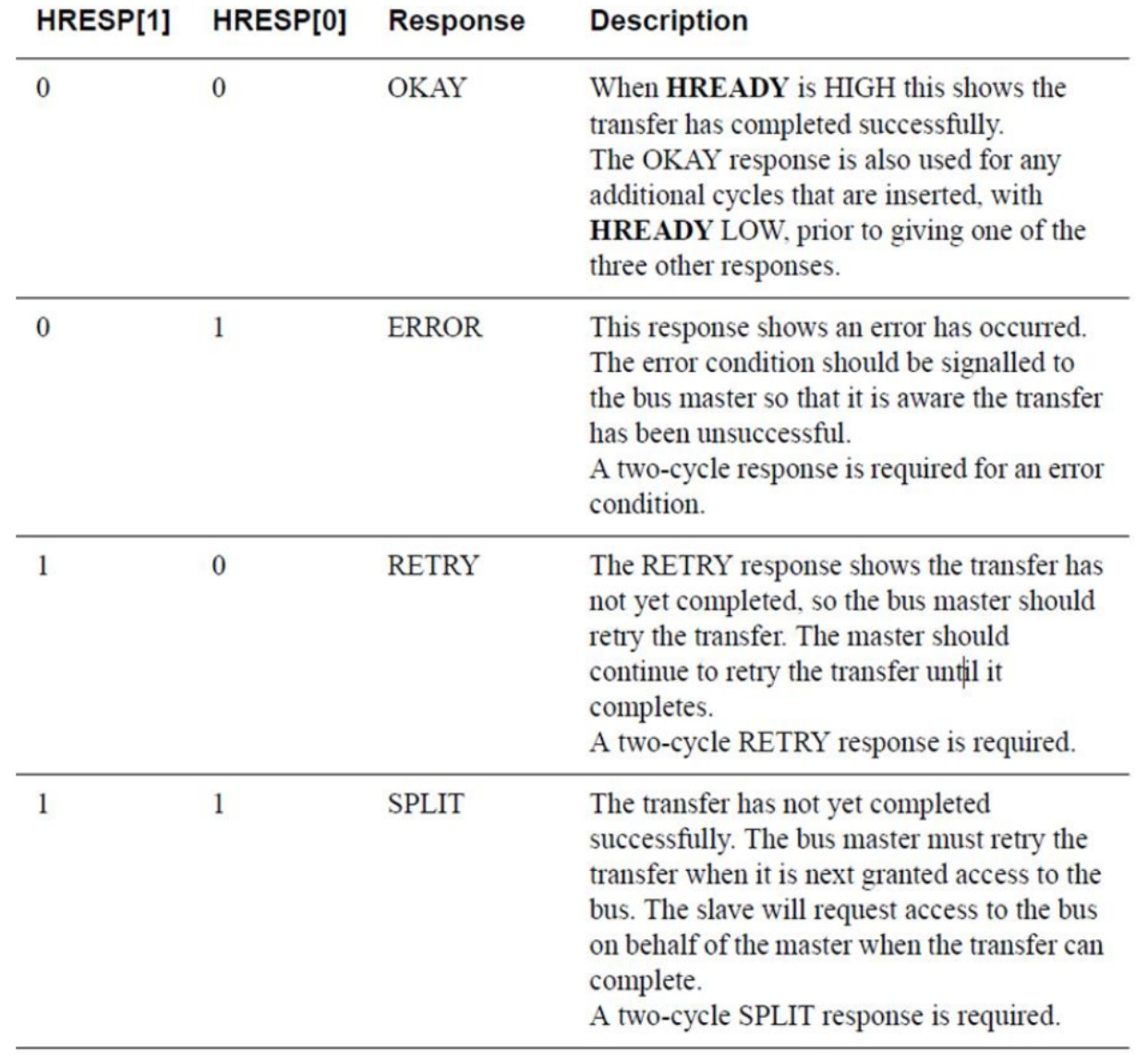#### 1.26 Assume A[3:0]=4’b0101,B[3:0]=4’b1111,which statement is correct()?

A. if C=&(A|B),then C=1’b0.
B. if C=(|A)&(|B),then C=1’b1.
C. if C=|(A&B),then C=1’b0.
D. if C=(&A)|(&B),then C=1’b0.

#### 1.27 For a truth table like below,which kind of logic cell could it be?

input A   input B   output Z
110
101
011
001

A. OR.
B. NAND.
C. XOR.
D. NOR.

#### 1.28 Choose the correct equation for power calculation()

A. Ptotal=Pstatic+Pdynamic.
B. Ptotal=Pleakage+Pinternal+Pswitching.
C. Ptotal=Pinternal+Pdynamic.
D. Ptotal=Pleakage+Pswitching.

#### 1.29 We need to define clock specifications in SDC file,using commands like below:create_clock -name GFXCLK -period 600 -waveform {0 300}.What can we know from this command?

A. Clock frequency.
B. Clock duty cycle.
C. Clock source latency.
D. Clock name.

#### 1.30 Which is the correct method to fix EM issue?

A. downsize victim driver.
B. double net width,double net spacing,chanfe route layer.
C. insert buffer in victim net.

A. 增益和带宽都大.
B. 增益和带宽都小.
C. 增益变大，带宽变小.
D. 增益变小，带宽变大.

#### 1.33 Ubuntu的内核有哪几个子系统（）

A. 进程管理系统.
B. 用户管理系统.
C. I/O管理系统.
D. 文件管理系统. E. 内存管理系统. F. 安全管理系统.

### 2 大疆

#### 2.1 下列关于多bit数据跨时钟域的处理思路，错误的有（）

A. 发送方给出数据，接收方用本地时钟同步两排再使用.
B. 发送方把数据写到异步fifo，接收方从异步fifo里读出.
C. 对于连续变化的信号，发送方转为格雷码发送，接收方收到后再转为二进制.
D. 发送方给出数据，发送方给出握手请求，接收方收到后回复.

#### 2.2 对12.918做无损定点化，需要的最小位宽是多少位，位宽选择11位时的量化误差是多少？

A. 12位，0.0118.
B. 13位，0.0039.
C. 12位，0.0039.
D. 13位，0.0118.

12用二进制表示需要4位，若小数用8位表示0.918/(2^(-8))=235.008，即235×2^(-8)=0.918，所以最少只要12位。当11位时，小数的位宽只有7,0.918/(2^(-7))=117.5040，117×2^(-7)=0.9141,0.918-0.9141=0.0039。

#### 2.3 考虑如下的4×4的“二维仲裁器”，R00到R33为输入，G00到G33为输出，N和W也为输入，E和S也为输出，假设所有的逻辑门（包括非门/与门/反相器）延时都为1ns，请问该电路的最大延迟为：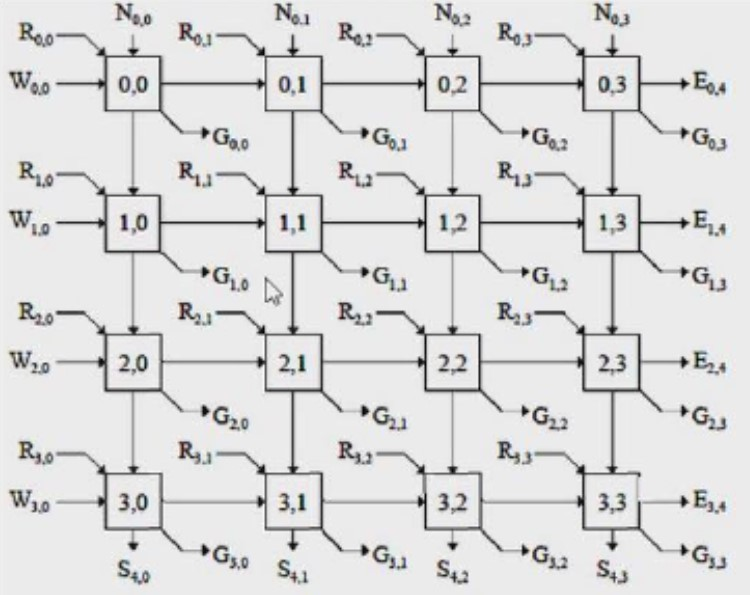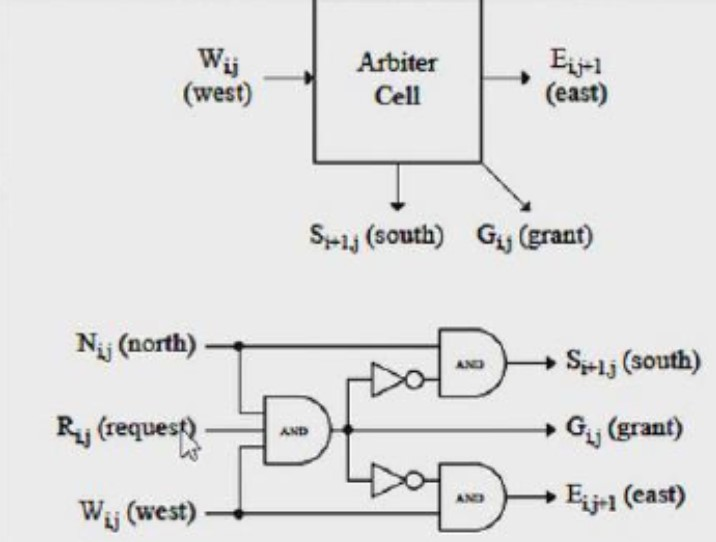A. 23ns.
B. 25ns.
C. 19ns.
D. 21ns.

#### 2.4 下图所示4位右移位寄存器，0时刻ABCD初始状态为0111，请写出5个时刻后的ABCD输出：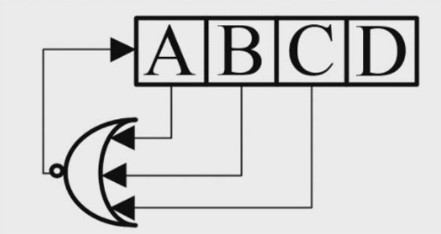A. 1010.
B. 0100.
C. 1101.
D. 1110.

TimeABCDNAND
001110
100110
200011
310000
400000
501100

#### 2.5 关于流水线设计的理解，错误的是：

A. 流水线设计会消耗较多的组合逻辑资源.
B. 流水线设计会导致原有通路延时增加.
C. 流水线设计的思想，是使用面积换取速度.
D. 关键路径中插入流水线，能够提高系统时钟频率.

#### 2.6 下列哪种逻辑门可以实现逻辑（A XOR B）OR （C AND D）？

A. NAND.
B. NOR.
C. XOR.
D. INV.

（A XOR B）OR （C AND D） = AB’ + A’B + CD
= ((AB’)’(A’B)’(CD)’)’
= ((A(BB)’)’((AA)’B)’(CD)’)’

#### 2.7 关于DFT的描述错误的是：

A. DFT测试不能覆盖电路时序问题.
B. DFT测试过程通常会消耗大量的动态功耗.
C. DFT的主要目的是发现芯片生产过程中出现的缺陷.
D. 寄存器扫描链是一种常用的DFT技术.

DFT的at-speed test会采用芯片PLL高速时钟进行寄存器的setup和hold测试

#### 2.8 下列那个工具不具备逻辑综合功能

A. Synplify.
B. Design Compiler.
C. Modelsim.
D. ISE.

#### 2.9 对芯片静态功耗影响最大的是哪一项

A. 工作模式.
B. 频率.
C. 负载.
D. 电压.

Pstat = Istat × Vdd

A. 电路中有多个输出.
B. 电路未达到最简.
C. 逻辑门类型不同.
D. 电路中的延时.

#### 2.11 指令系统中程序控制类指令的功能是

A. 实现主存于CPU之间的数据传送.
B. 实现程序执行顺序的改变.
C. 实现算术和逻辑运算.
D. 时间堆栈操作.

• 跳转指令；
• 循环指令；
• 子程序指令；
• 中断指令。 这些指令可以控制程序的执行顺序。

A. 高通，抗周期延拓.
B. 高通，抗混叠滤波.
C. 低通，抗周期延拓.
D. 低通，抗混叠滤波.

A. 2.
B. 4.
C. 3.
D. 1.

#### 2.14 如下关于“线与”逻辑的描述，错误的是：

A. 可以使用OC门来实现“线与”.
B. “线与”逻辑必须在输出端加一个下拉电阻.
C. “线与”逻辑是两个输出信号相连可以实现“与”的功能.
D. 可以用OD门来实现“线与”.

OD：开漏，是对MOS管而言；
OC：集电极开路，是对双极性管而言。

#### 2.15 关于异步处理，以下说法正确的是：

A. 静态配置信号可以不做异步处理.
B. 异步FIFO采用格雷码的原因是为了提高电路速度.
C. 异步处理需要考虑发送和接收时钟之间的频率关系.
D. 单比特信号打两拍之后可以避免亚稳态的发生.

#### 2.16 关于16点的FFT描述正确的是：

A. 每个蝶形算法需要一次复数加法.
B. 每级有8个蝶形算法.
C. 每个蝶形算法需要一次复数乘法.
D. 共有4级分解.

#### 2.17 在不增加pipeline的情况下，如何解决一条critical path的setup时序不满足的问题

A. 使用更先进工艺的工艺库.
B. 在这条path上插入寄存器.
C. 将部分组合逻辑电路搬移到前级path上.
D. 降低时钟频率.

#### 2.18 下列关于代码覆盖率描述错误的是：

A. 代码覆盖率达到百分之一百说明代码bug已消除.
B. 代码覆盖率包括功能覆盖率.
C. 代码覆盖率包括条件覆盖率.
D. 代码覆盖率包括语句覆盖率.

• 行覆盖率；
• 条件覆盖率；
• 状态机覆盖率；
• 翻转覆盖率。 功能覆盖率反映开发出来的需要覆盖的功能点覆盖的比例。 断言覆盖率测量断言被触发的频繁程度。

#### 2.19 C语言中定义了一个全局数组，编译后此数组可能分配在下列哪个阶段？

A. Text段.
B. Bss段.
C. Data段.
D. Stack段.

BSS存放的是未初始化的全局变量；
DATA存放的是初始化的全局变量。

A. 资源共享.
B. 关键路径优化.
C. 流水线.
D. 串行化.

#### 2.21 对于PSK和QAM调制，以下哪些说法是正确的？

A. 对于QAM调制，星座图的每个点的幅度相等.
B. 2PSK和4PSK在归一化条件下，其幅值都是1.
C. 4-QAM调制与QSPK的调制方式一致.
D. 在同样信道条件下，16-QAM的误码率大于4-QAM.

PSK调制，星座图中的点都位于单位圆上，模相同（都为1），只有相位不同。而QAM调制星座图中的点不再位于单位圆上，而是分布在复平面的一定范围内，各点如果模相同，则相位必不相同，如果相位相同则模必不相同。

#### 2.22 wirte-back cache和write-through cache的区别：（write-back）cache只在cache line被替代的时候把cache里的有效数据写下一级存储。

write-through（直写模式）在数据更新时，同时写入缓存cache和后端存储。此模式的优点是操作简单，缺点是因为数据修改需要同时写入存储，数据写入速度较慢。
write-back（回写模式）在数据更新时写入缓存cache只在数据被替换出缓存时，被修改的缓存数据才会被写到后端存储。此模式的优点是数据写入速度快，因为不需要写存储；缺点是已更新后的数据未被写入存储时出现系统掉电的情况，数据将无法找回。

#### 2.24 FPGA时序检查中对于异步复位电路的时序分析分别叫（恢复时间检查）和（移除时间检查）。

recovery time：恢复时间。

removal time：移除时间。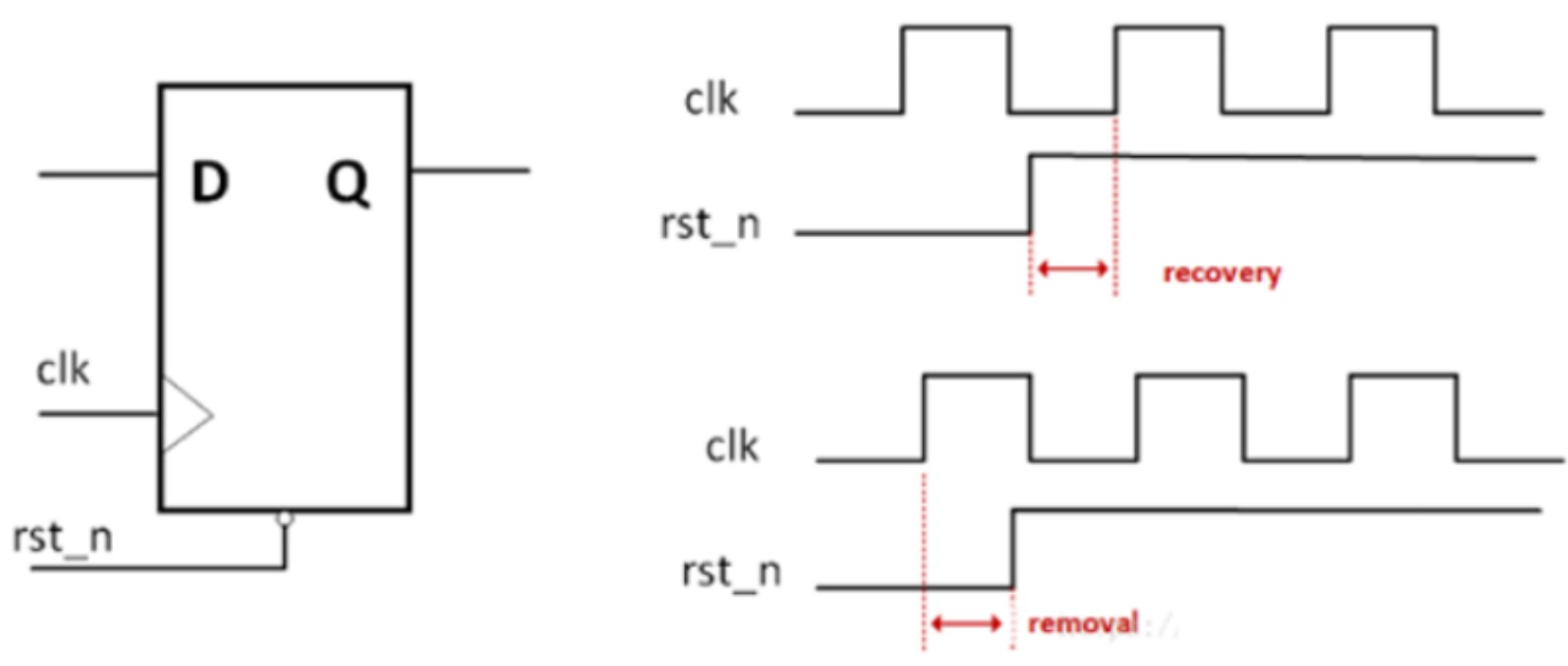#### 2.25 并行计算是提高程序速度的关键，设a为并行计算部分所占比例，n为并行处理的节点数，则并行计算带来的加速比为（1 /（a/n + 1 - a））。

S = （Ws + Wp）/（Ws + Wp/p），Ws为程序中串行部分，Wp为程序中的并行部分，p为并行节点数。

#### 2.26 下图球框由三个相同的铁圈两两正相交组成，每个铁圈的电阻均为R。AB两点间的电阻为（5R/48）。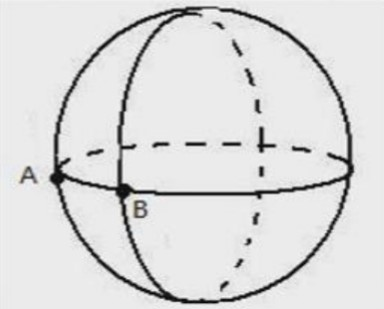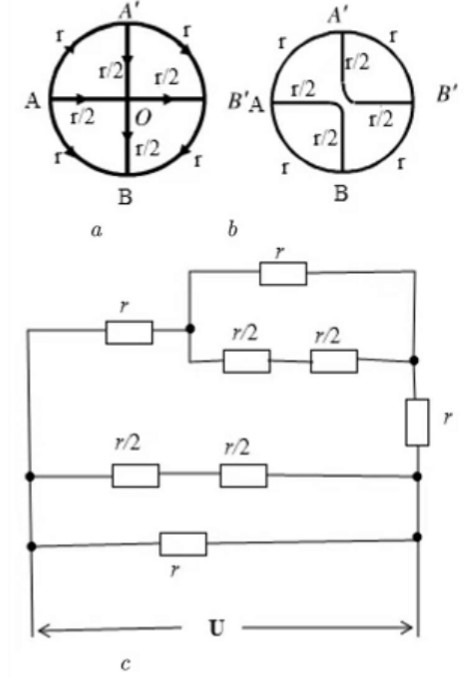#### 2.27 signal_a是clk_a(300M)时钟域的一个单时钟脉冲信号，如何将其同步到时钟域clk_b(100M)中，并产生出signal_b同步脉冲信号。请用verilog代码描述，并画出对应的时序波形说明图。

``````module div_3_50(
input   clk,
input   rst_n,

input   in,

output  q,
output  out,
);

reg q1,q2;
reg [1:0] count1,count2;

reg in_p,in_pp,in_ppp;

wire out_b;

reg out_bb,out_bbb;

assign q=q1|q2;

always_ff@(posedge clk , negedge rst_n)begin
if(!rst_n) begin
q1 <= '0;
count1 < ='0;
end
else if(count1==0) begin
q1 <= ~q1;
count1 <= count1 + 1;
end
else if(count1==1) begin
q1 = ~q1;
count1 <= count1 + 1;
end
else begin
count1 <= '0;
end
end

always_ff@(negedge clk , negedge rst_n)begin
if(!rst_n) begin
q2 <= '0;
count2 < ='0;
end
else if(count2==0) begin
q2 <= ~q2;
count2 <= count2 + 1;
end
else if(count2==1) begin
q2 = ~q2;
count2 <= count2 + 1;
end
else begin
count2 <= '0;
end
end

always_ff@(posedge clk , negedge rst_n)begin
if(!rst_n) begin
in_p   <= '0;
in_pp  <= '0;
in_ppp <= '0;
end
else begin
in_p   <= in;
in_pp  <= in_p;
in_ppp <= in_pp;
end
end

assign out_b = in_ppp|in_pp|in_p;

always_ff@(posege clk , negedge rst_n)begin
if(!rst_n) begin
out_bb  <= '0;
out_bbb <= '0;
end
else begin
out_bb  <= out_b;
out_bbb <= out_bb;
end
end

assign out = out_bbb;

endmodule

``````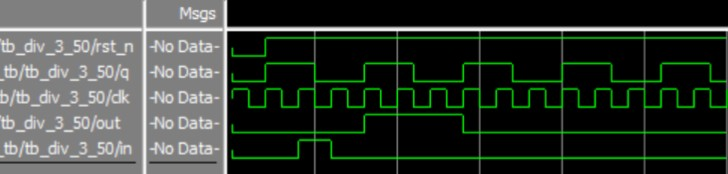#### 2.28 如下图，一个高速接口的端口电路示意图，要求D端发送数字0/1。D/Q端收到相同的数字0/1。VREF电压为比较器数字输出0/1的判决电压。

（1）S1断开时，DQ端VREF电压需设置为？
（2）S1连通时，DQ端VREF电压需设置为？
（3）驱动端发送0时功耗比较低，这句话正确吗？为什么？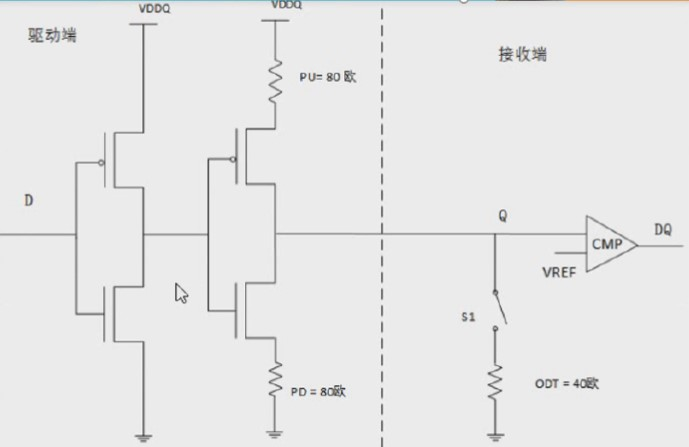（1）S1断开时，若D为1，Q端电压为VDDQ，因此VREF要小于VDDQ。若D为0，Q端电压为0，因此VREF要大于0，因此0<VREF<VDDQ。
（2）S1连通时，若D为1，Q端电压为VDDQ/3。若D为0，Q端电压为0，因此VREF要大于0，因此0<VREF<VDDQ/3。
（3）驱动端为0时，NMOS管道通，反相器输出接地，电阻没有电流，因此功耗较低。

#### 2.29 用Moore型状态机实现序列“1101”从右至左的不重叠检测。

（1）请画出状态转换图，其中状态用S0,S1,S2…表示。
（2）针对这个具体设计如何衡量验证的完备性？

（1）：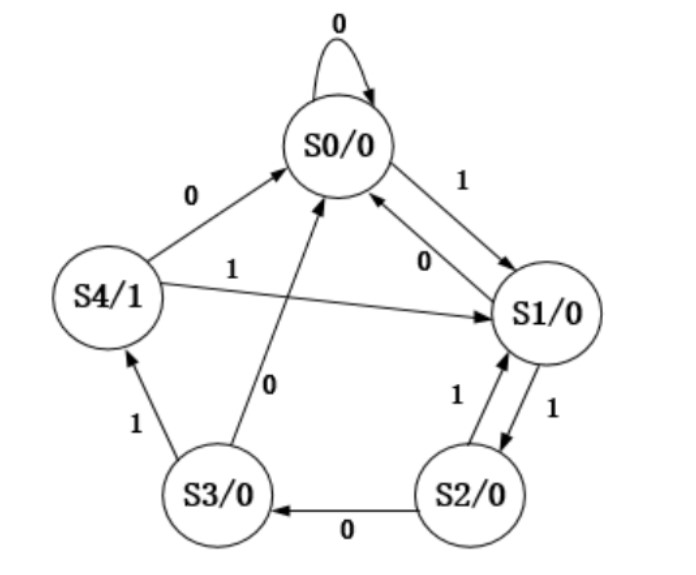（2）：可以从定向测试，随机测试两种方式中产生激励，从代码覆盖率的角度保证代码覆盖率达100%。

#### 2.30 进行一个运算单元的电路设计，A[7:0]*11111011，尽量用最少的资源实现，写出对应的RTL代码。

``````module mul(
input  [7:0]    A,
output [15:0]   B
);

wire [15:0] reg1;
wire [15:0] reg2;
wire [15:0] reg3;

assign reg1 = {A,{8{1'b0}}};
assign reg2 = ,A,{2{1'b0}}};
assign reg3 = ,A};

assign B = reg1 - reg2 - reg3;

endmodule
``````

### 3 联芸科技

#### 3.1 请解释D触发器和Latch的区别，解释同步复位和异步复位的区别及优缺点。

• Latch有电平触发，非同步控制。在使能信号有效时Latch相当于通路，在使能信号无效时Latch保持输出状态。D触发器由时钟沿触发，同步控制。
• Latch容易产生毛刺，D触发器则不易产生毛刺。
• 如果使用门电路来搭建Latch和D触发器，则Latch消耗的门资源比D触发器要少，这是Latch比D触发器优越的地方。所以在ASIC中使用Latch的集成度比D触发器高，但在FPGA中恰好相反，因为FPGA中没有标准的Latch单元，但有D触发器单元，一个Latch需要多个LE才能实现。
二、
• 同步复位：同步复位只有在时钟沿到来时复位信号才起作用，则复位信号持续时间应该超过一个时钟周期才能保证系统复位。
• 异步复位：异步复位只要有复位信号系统马上就复位，因此异步复位抗干扰能力差，有些噪声也是能系统复位，因此有时候显得不够稳定，要想设计一个好的复位最好使用异步复位同步释放。
• 同步复位：
• 优点：
• 有利于仿真器仿真；
• 可以使用所设计的系统成为100%同步时序电路，这大大有利于时序分析，而且综合出来的fmax一般较高。
• 因为他只有在时钟有效电平到来时才有效，所以可以滤掉高于时钟频率的毛刺。
• 缺点：
• 复位信号的有效时长必须大于时钟周期，才能真正被系统识别并完成复位任务。同时还要考虑，诸如：clk skew，组合逻辑路径延>时，复位延时等因素；
• 由于大多数的逻辑器件的目标库内的DFF都只有异步复位端口，所以，倘若采用同步复位的话，综合器就会在寄存器的寄存器的数据输入端口插入组合逻辑，这样就会耗费较多的逻辑资源。
• 异步复位：
• 优点：
• 大多数目标器件库的DFF都有异步复位端口，因此采用异步复位可以节省资源；
• 设计相对简单；
• 异步复位信号识别方便，而且可以很方便的使用FPGA的全局复位端口GSR。
• 缺点：
• 在复位信号释放（release）的时候很容易出现问题，倘若复位释放时恰好在时钟有效沿附近，就很容易使寄存器输出出现亚稳态，从而导致亚稳态。
• 复位信号容易收到毛刺影响。

#### 3.2 解释什么叫clock gating？并说明一下通常情况下为什么要做clock gating？简单列举通常实现的方法有哪些？

clk信号和clk_enable相与。

#### 3.3 实现一个可以1-8分频任意切换的分频器，要求无论是奇数分频还是偶数分频，分频后的时钟的duty cycle都是50%。

``````module Duty_Cycle#(
parameter   HIGH_TIME = 1,  //高电平时间
LOW_TIME  = 2,  //低电平时间
BIT_WIDTH = 2   //位宽
)(
input       clk,            //时钟
input       rst_n,          //异步复位

output reg  clk_out         //输出
);

reg [BIT_WIDTH-1:0] count;  //计数器

always_ff @(posedge clk, negedge rst_n) begin
if(!rst_n) begin
count <= '0;
end
else if(count < HIGH_TIME) begin
count <= count + 1;
clk_out <= '1;
end
else if((count < HIGH_TIME+LOW_TIME-1) && (count >= HIGH_TIME))begin
clk_out <= '0;
count <= count + 1;
end
else if(count >= HIGH_TIME+LOW_TIME-1)begin
clk_out <= '0;
count <= '0;
end
else begin
clk_out <= clk_out;
count <= count;
end
end

endmodule
``````

``````module Frequency_Divider#(
parameter   DIV_COEFF = 3,  //分频系数
BIT_WIDTH = 2   //分频系数位宽
)(
input       clk,            //时钟
input       rst_n,          //复位

output      clk_out         //分频时钟
);

//======================================================
//奇偶判断，0为偶

wire odd_even;
wire [BIT_WIDTH-1:0] div_coeff;

assign div_coeff = DIV_COEFF;
assign odd_even = div_coeff;

//======================================================
//计算高低占空比时间

parameter HIGH_TIME = DIV_COEFF/2;
parameter LOW_TIME  = DIV_COEFF-HIGH_TIME;

//======================================================
//根据奇偶分频分配时钟
reg clk_p,clk_n;

always_comb begin
if(odd_even)begin
clk_p = clk;
clk_n = ~clk;
end
else begin
clk_p = clk;
clk_n = clk;
end

end

wire clk_out_p,clk_out_n;

Duty_Cycle#(
.HIGH_TIME (HIGH_TIME),
.LOW_TIME  (LOW_TIME),
.BIT_WIDTH (BIT_WIDTH)
)u1_Duty_Cycle(
.clk       (clk_p),
.rst_n     (rst_n),

.clk_out   (clk_out_p)
);

Duty_Cycle#(
.HIGH_TIME (HIGH_TIME),
.LOW_TIME  (LOW_TIME),
.BIT_WIDTH (BIT_WIDTH)
)u2_Duty_Cycle(
.clk       (clk_n),
.rst_n     (rst_n),

.clk_out   (clk_out_n)
);

assign clk_out = clk_out_p | clk_out_n;

endmodule
``````

#### 3.4 请画一个状态机（状态转换图）来检测串行比特流中的‘10011’。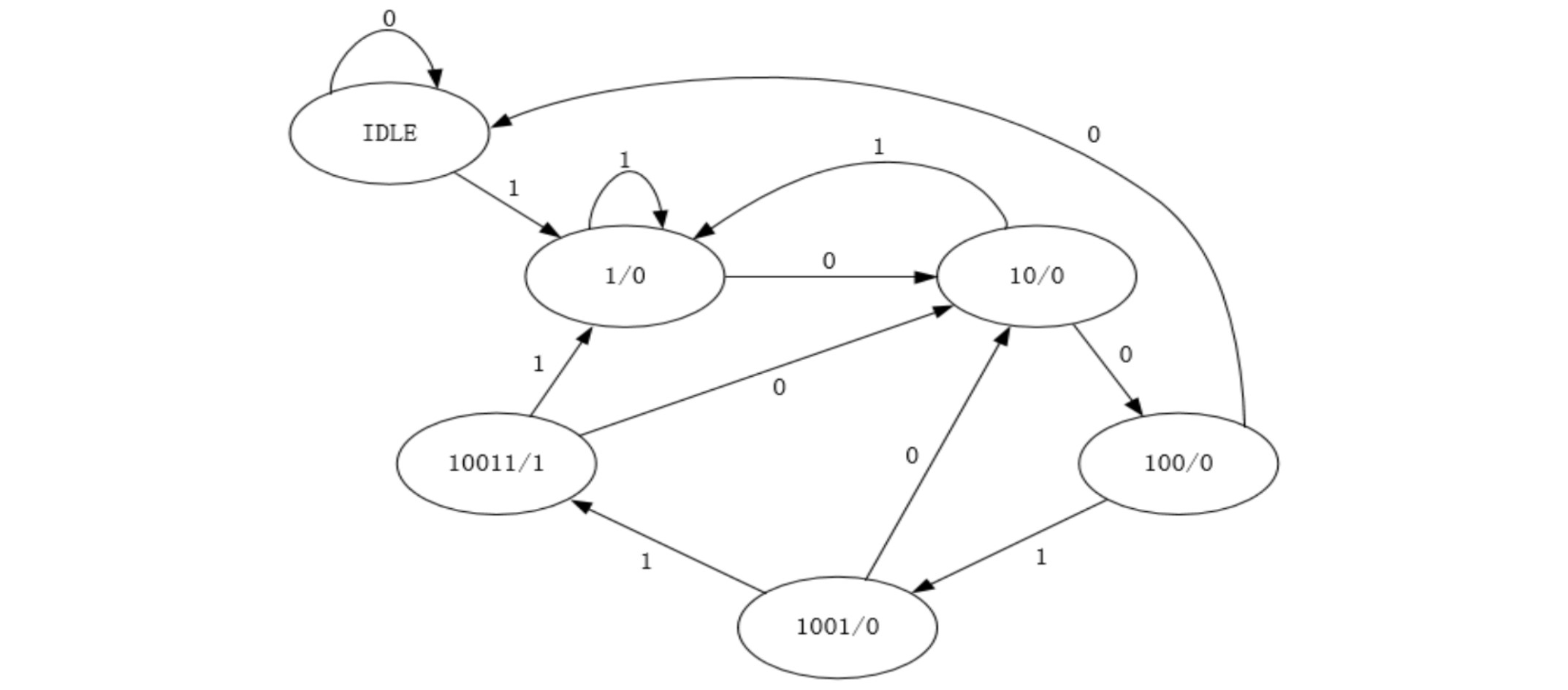#### 3.5 现有三个输入信号分别是a，b，c，并且前级DFF Clock到a，b，c的延时分别是Ta，Tb，Tc（Ta>Tb>Tc），请用两个二输入选择器对a，b，c实现三输入选择输出，画电路图并解释设计思路，不需要考虑Clock Skew的影响。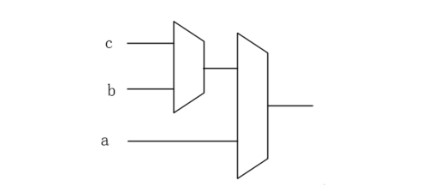#### 3.6 请画出下列数字电路D触发器输出Q0，Q1的波形图（复位之后Q1，Q2均为0，画出复位后8个时钟周期）。

Q1   Q2
RESET00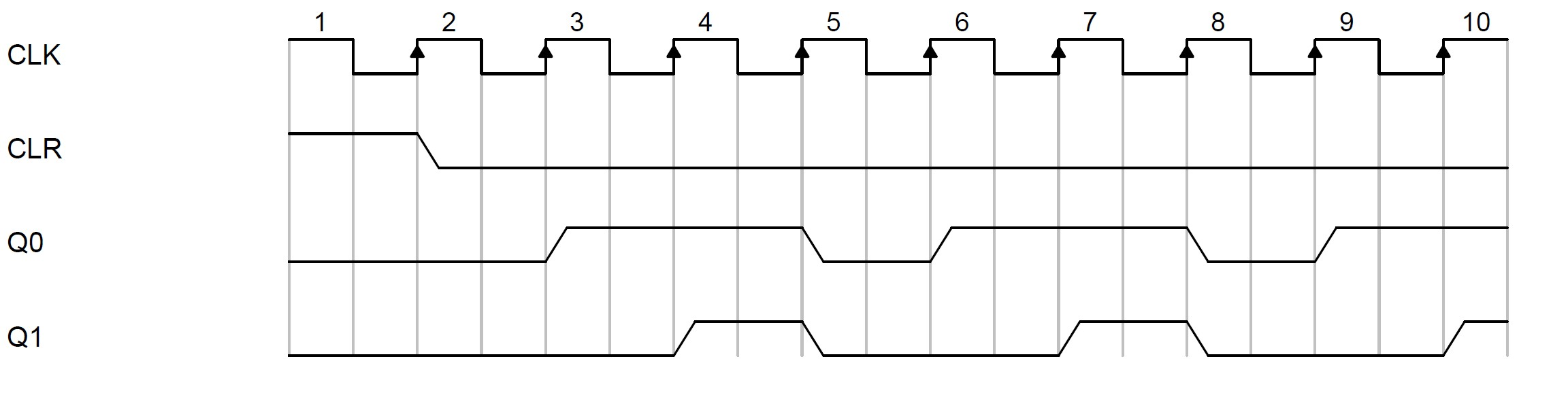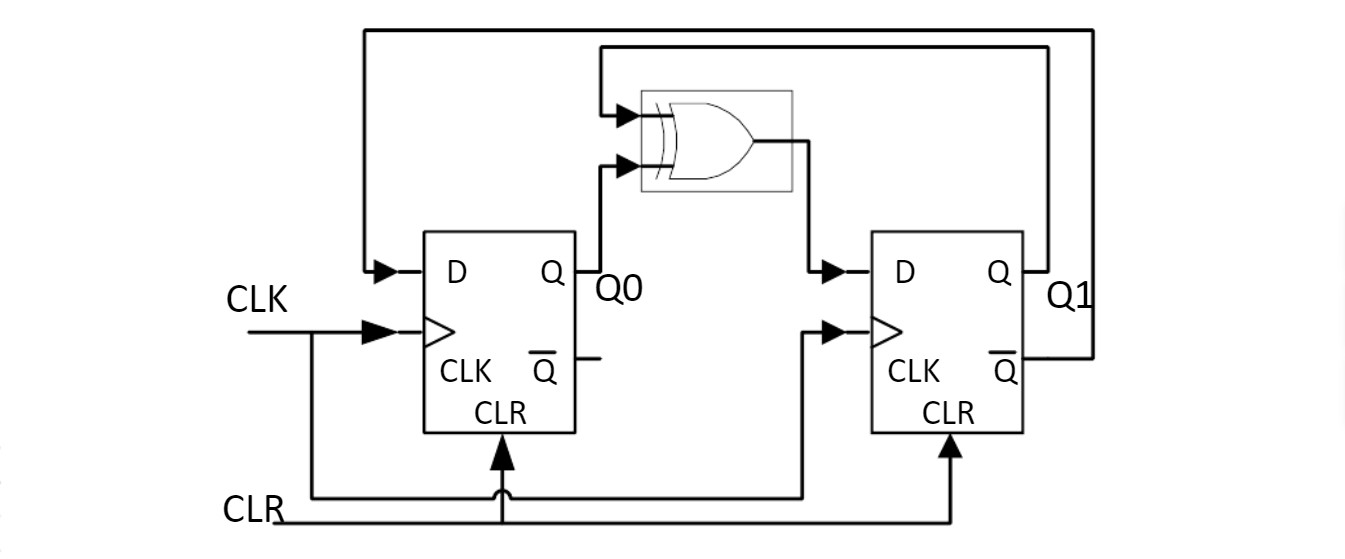#### 3.7 分析代码覆盖率时，verilog语句if(a||b&&c)有那几个条件需要覆盖？请用表格列出每种状况下a/b/c的值（a/b/c均为bit类型，如果是0或1都无所谓，请用“-”表示）。

a  b  c  a||b&&c
1--1
0000
0010
0100
-111

a为真或b&&c为真，结果为真；
a和b&&c都为假时，结果为假。

。。。

#### 3.9 使用C或Verilog/SystemVerilog，定义并实现一个函数，传递三个int类型参数A，B，C，函数返回后，A的值不变，B的值变为A+B，C的值变为A-C，请写出函数原型定义与函数体的实现。

``````module A;

int A;
int B;
int C;

initial begin
A=1;
B=2;
C=3;

\$display("%d,%d,%d",A,B,C);
#1;

func1(A,B,C);

\$display("%d,%d,%d",A,B,C);
end

function automatic viod func1(ref int A,ref int B,ref int C);
A=A;
B=A+B;
C=A-C;
endfunction
endmodule
``````

#### 3.10 请列出下述模块与中断先关的所有功能测试点。

中断使能寄存器reg_int_enable[7:0];

1. APB总线对三个寄存器任意一位读写操作功能测试；
2. reg_int_status寄存器任意一位W1C功能测试；
3. APB总线配置reg_int_enable寄存器任意一位为1，对应位status置位功能测试；
5. reg_int_status寄存器所有位W1C操作，信号interrupt拉低功能测试。

### 4 联发科

#### 4.1 ASIC流程，说出5个以上环节；Verilog说出5个以上keyword。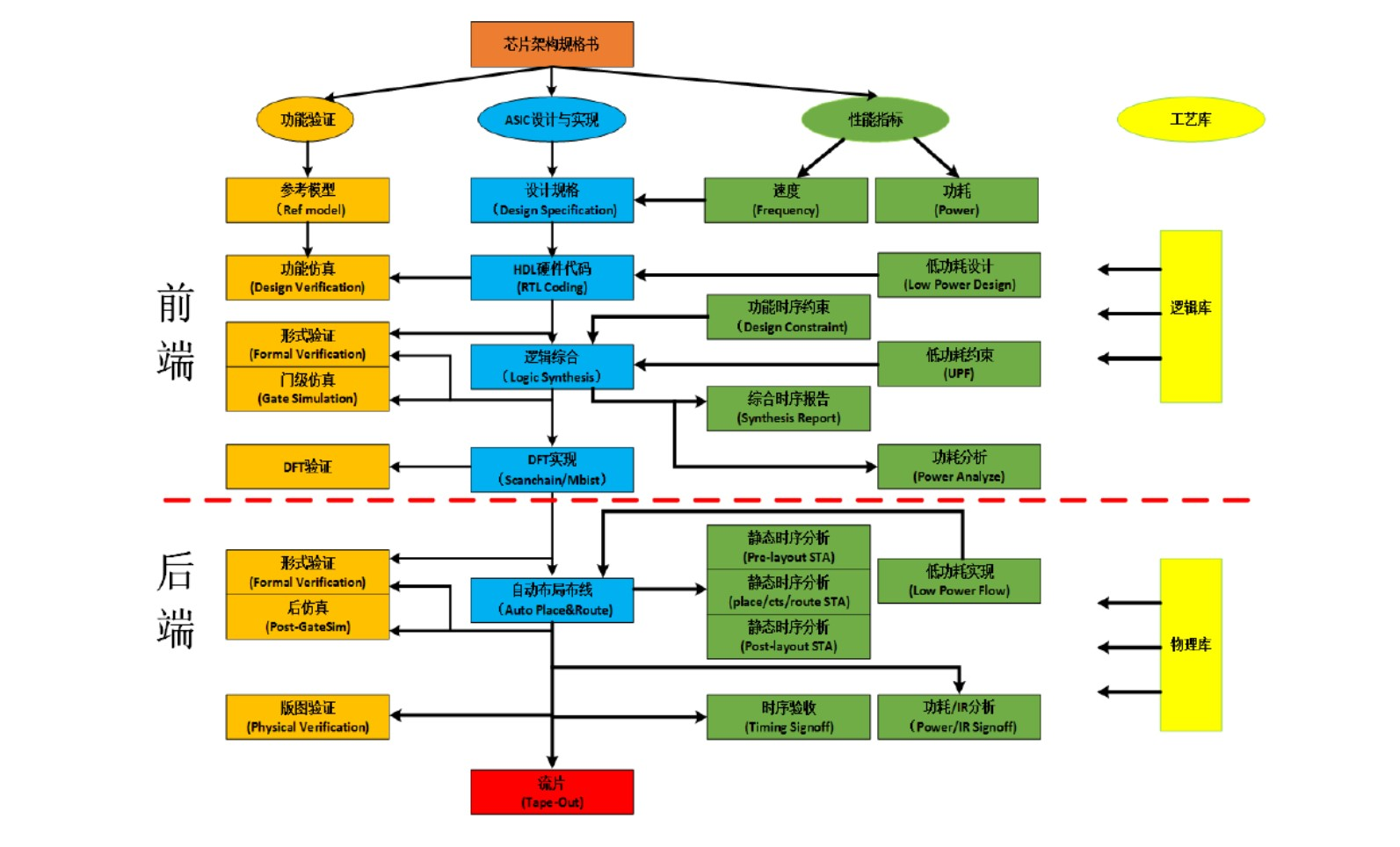#### 4.2 用一个mux和一个反相器实现xor，画电路或Verilog代码实现。

ABXOR
000
011
101
110#### 4.3 如下图所示：clk到Q的输出延时为T1min<T1<T1max，组合逻辑的延时为：T2min<T2<T2max，时钟周期为T。满足约束条件下，计算寄存器的setup和holdup。T1max + T2max + Tsetup < T；

T1min + T2min > Tholdup。

#### 4.4 如下图所示：根据A的波形，画出B，C的波形，寄存器是同步清零的。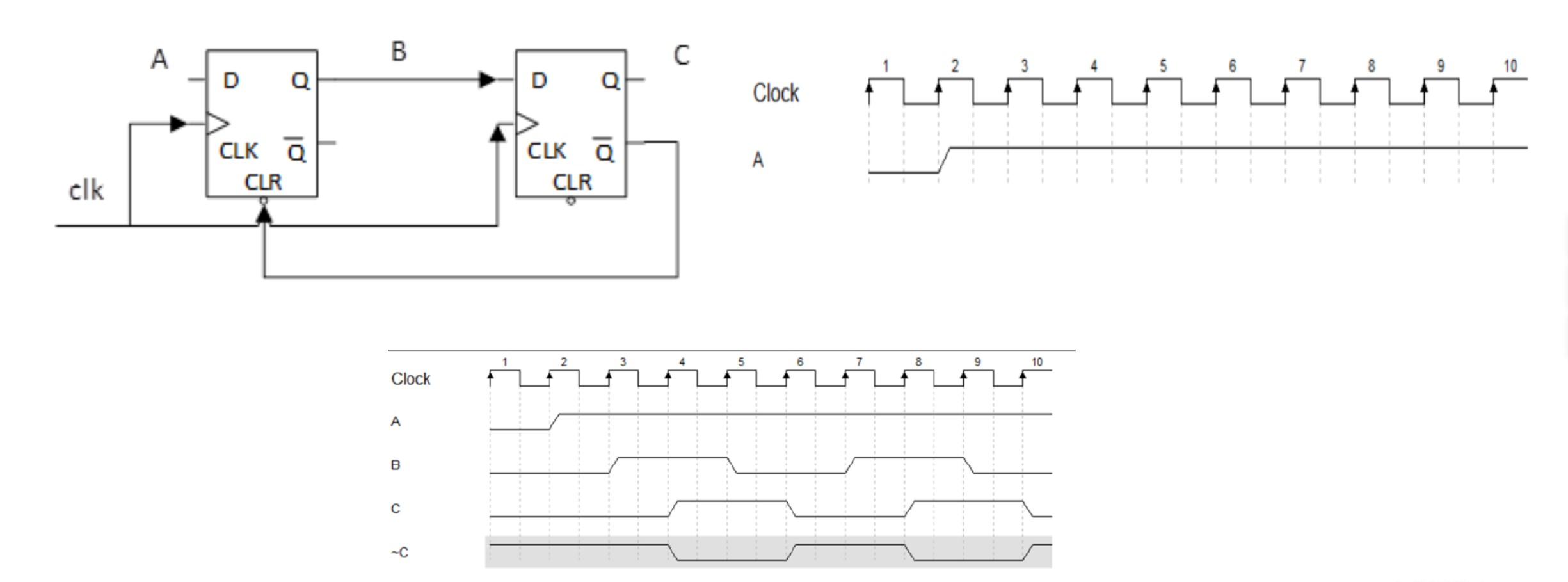#### 4.5 十进制的12.8125用二进制的数表示，十六进制的A8D6用八进制的数表示。

12 = 1100;
0.1825 × 4 = 3.25，表示：11.01，所以0.8125=0.1101；

A8D6 = 1010100011010110 = 124326。

#### 4.6 SV比Verilog强在哪里？OVM、UVM、VMM是什么？

SV支持面向对象的编程，支持断言的语法，支持更多的数据类型。 VMM：Verification Methodology Manual，由ARM和Synopsys提出的，寄存器解决方案RAL机制；

#### 4.8 int b = 100；int *a； a=&b有什么意义？a=b有什么意义？

a=&b定义指针变量a，变量指向整形数据b的地址，printf(“%d”,a)结果为100；
a=b表示指针变量a的值为100，
a表示一个地址为100的数据。

#### 4.10 CPU cache miss/hit 区别

CPU要访问的数据在Cache中有缓存，称为“命中”（Hit），反之则称为“缺失”（Miss）。

#### 4.11 110序列选择器，画出状态图，写出Verilog代码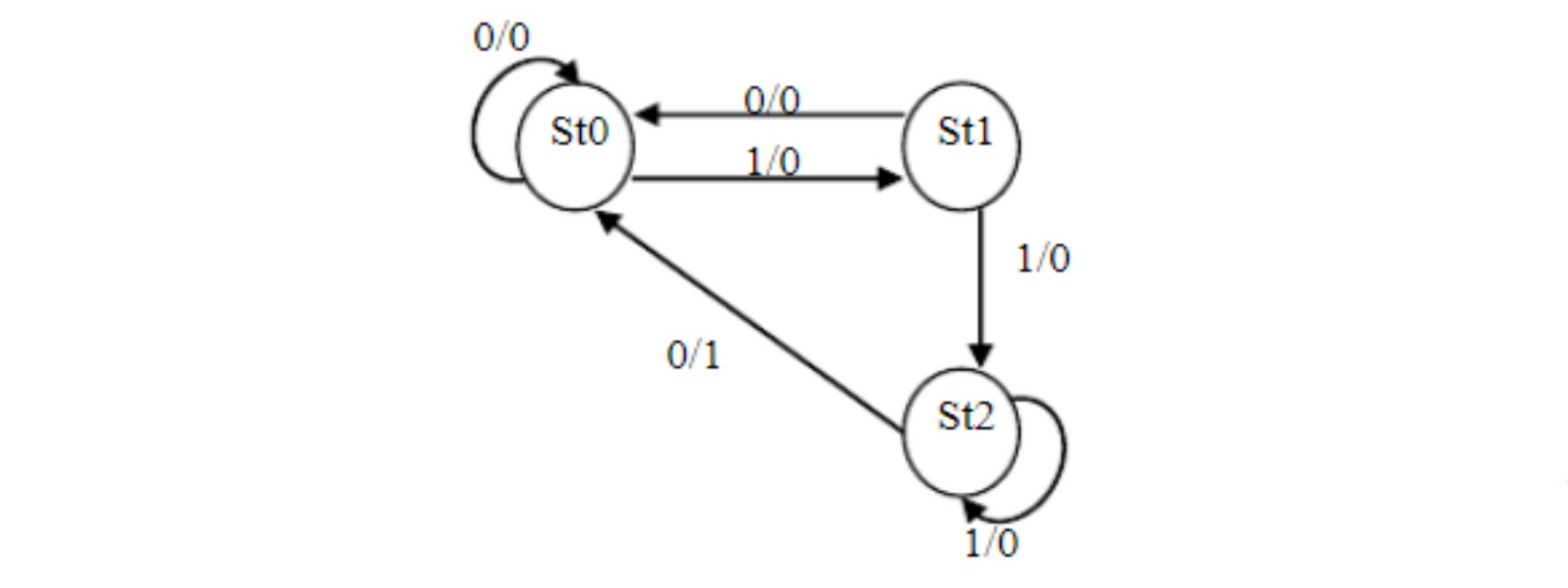``````module Sequential_Detector(
input       clk,
input       rst_n,

input       in,
output      out
);

parameter   s0 = 2'b00,
s1 = 2'b01,
s2 = 2'b10;

reg [1:0] cur_state,next_state;

reg out_r;

always_ff(posedge clk,negedge rst_n)begin
if(!rst_n)
cur_state <= s0;
else
cur_state <= next_state;
end

always_comb begin
case(cur_state)
s0: next_state = (in==1'b1)?s1:s0;
s1: next_state = (in==1'b1)?s2:s0;
s2: nexe_state = (in==1'b0)?s0:s2;
default: nexe_state = s0;
endcase
end

always_ff@(posedge clk,negedge rst_n)begin
if(!rst_n)
out_r <= '0;
else begin
case(cur_state)
s0: out_r <= '0;
s1: out_r <= '0;
s2: begin
if(in==0)
out_r <= '1;
else
out_r <= '0;
end
endcase
end

assign out = out_r;

endmodule
``````

#### 4.12 某夜，A、B、C、D四人要过桥，该桥每次只能通行2个人，只有一个手电筒，过桥必须持有手电筒，A、B、C、D四人单独过桥的时间分别为1分钟、2分钟、5分钟和10分钟。现要求四人最短时间完成过桥，不能折返，请问该如何操作？

AB先过去，A返回，CD再过去，B返回，AB再走一遍：2+1+10+2+2=17min。

### 5 平头哥

#### 5.1 SV里四值变量有哪些？

• reg（单比特或多比特的无符号数）；
• wire（线网）；
• time（64比特的无符号数）；
• integer（32比特的有符号数）；
• logic（SV改进型reg）。

#### 5.2 SV里面动态数组、关联数组、队列各自的优缺点，应用场合。

• 特点：
• 动态数组：可以在仿真时分配空间或者调整宽度，这样在仿真中就可以使用最小的存储量；
• 关联数组：用来保存稀疏矩阵的元素，当随一个非常大的地址空间进行寻址时，SV只对实际写入的元素分配空间，比定宽和动态数组所> 占用的空间要小得多；
• 队列：结合了链表和数组的优点，可以在队列的任意位置增加或删除元素，这类操作在性能上比动态数组小得多，可以通过索引对任意> 元素进行访问；
• 应用场合：
• 动态数组：随机事务不确定位宽的大小；
• 关联数组：需要建立一个超大容量数组，用关联数组来存放稀疏矩阵中的元素；
• 队列：增加元素或删除元素方便。

#### 5.4 数据的类型转换怎么做？静态强制类型转换绝动态强制类型转换有什么区别？

• 静态转换：转换时指定目标类型，并在需要转换的表达式前加上单引号即可；
• 动态转换：使用函数\$cast。
• 区别：静态类型转换操作不对转换值进行检查，具有一定的危险性；动态转换在运行时将进行检查，如果转换失败会产生运行时错误。

#### 5.5 virtual interface的作用

• interface只在module中声明，在class中要用virtual interface；
• virtual interface是仿真运行时才连接到DUT上的，如果只是interface，在编译时就必须进行连接，在class中时是automatic，在运行> 的时候产生；
• virtual interfacec主要完成接口的动态分配，只要在top层定义virtual interface，其它层不需要改变，直接通过interface传递参数> 即可；
• 消除绝对路径，避免修改的时候改的东西很多。

#### 5.6 多态

• 多态性（polymorphisn）是允许你将父对象设置称为一个和更多的他的子对象相同的技术，复制之后，父对象就可以根据当前赋值给它的子> 对象的特性以不同的方式运作。简单的说就是允许将子类类型的指针赋值给父类类型的指针。
• 多态的作用是为了实现接口重用，为了类在继承和派生的时候，保证使用“家谱”中任一类的实例的某一属性时的正确调用。
• 多态的实现方法：
• 覆盖：是指子类重新定义父类的虚函数的做法；
• 重载：是指允许存在多个同名函数，而这些函数的参数表不同（或许参数个数不同，或许参数类型不同，或许两者都不同）。

#### 5.14 fork join，fork join_any，fork join_none的用法

• fork join：当前速优子进程执行完毕，才会继续执行后面的进程；
• fork join_any：当前的任一子进程执行完毕后，才会继续执行后面的进程；
• fork join_none：当前的子进程并不阻塞后面的进程执行。

#### 5.15 阻塞赋值和非阻塞赋值的区别。

• 阻塞赋值的操作符号用等号（=）表示，当前语句的赋值阻塞其它语句的赋值；
• 非阻塞赋值的操作符号用小于等于好（<=）表示，当前语句的赋值不阻塞其它语句的赋值。

#### 5.16 Callback机制的运行机理

Callback机制，其实是使用OOP来实现的一种程序开发者向程序使用者提供的模块内部的接口，可以在Test_case的高度改变其他Component的一些行为。

• 派生一个uvm_callback的类；
• typedef定义一个uvm_callbacks pool；
• 在对应的组件中注册派生的callback类。 对于程序的使用者而言：
• 定义一个新的callback的派生子程序开发者所定义的类，并重写类的方法；
• 在对应的test_base中实例化并添加callback的类。

#### 5.17 clock模块为什么要放在top module中，不放在program中的原因？

SV介绍program的一个重要部分就是为了将涉及和验证的调度区域通过显式的方式来安排，例如设计部分被建议放置在module中，而测试采样部分被建议放置在program中，program中不能使用always，program中的仿真时间与RTL中的是有区别的，SV将同一仿真时刻分为四个区域，相当于在原verilog的基础上又为program增加了一个执行区间，一个采样区间，所以clk的定义不能放在program中。当program中的initial结束时，SV会调用\$finish完成仿真。

#### 5.18 谈谈对block level，chip level，product level验证的理解？

• block level：
• 每个模块验证首先要考虑的是哪些功能点是可以在模块一级完全验证的的，这基于如下考虑：
• 内部功能如状态机验证；
• 内部数据存储验证；
• 数据打包功能、编解码功能；
• 指令执行；
• 寄存器配置；
• 同时也需要考虑哪些功能无法在模块一级被验证到：
• 与其他相邻模块的互动信号；
• 与其他子系统的互动信号；
• 与芯片外部的互动信号；
• 与电源开关的验证。
• chip level：
• 在芯片系统级，验证平台的复用性较高，主要因为：
• 外围的验证组件不需要像模块级、子系统级的组件数量多且经常需要更新，它们主要侧重于验证芯片的输入输出；
• 芯片内部的子系统之间的交互、协作检查主要交给了处理器和子系统，从寄存器检查和数据检查入手，写直接测试用例。
• 在芯片系统级的验证侧重于不同子系统之间的信号交互问题，以及实现更贴近实际使用的用例。这里的实习用例并非是在系统软件层面的，而是将> 系统软件层面的场景进一步拆分为多个模块互动情景，再分类测试的。
• product level：
• 直接的软件测试激励，可根据示波器等工具观察波形变化，也可以根据SDK软件debug。

#### 5.20 UVM架构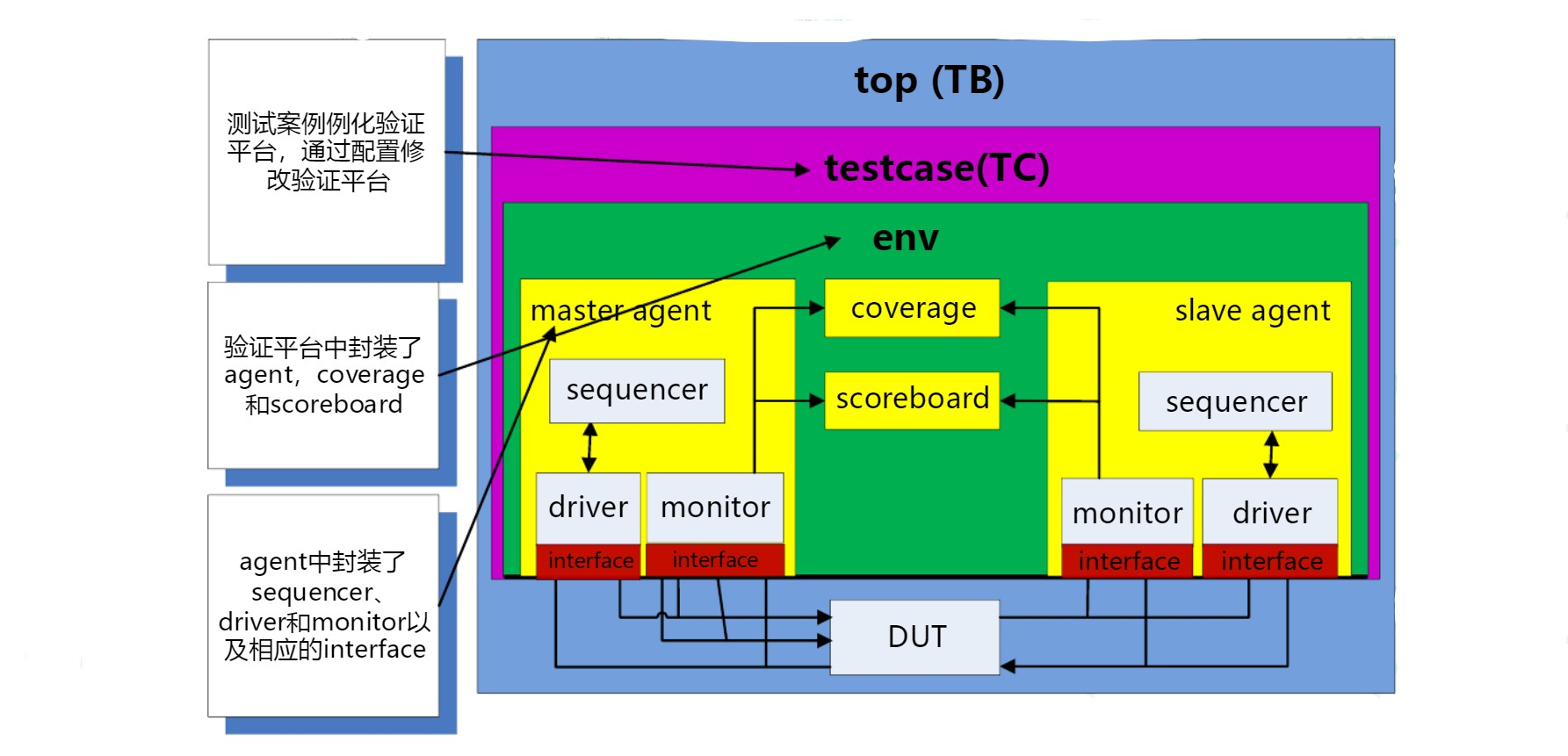#### 5.22 请用ROM实现以下Verilog代码对应的功能：

``````always@(posedge clk) begin
if(a&&b)
q <= 1'b0;
else
q <= !q;
end
``````

ROM查找表：

abDQ
0001
0010
0101
0110
1001
1010
1100
1110

#### 5.23 设计一个时序电路，输入是2个8bit的无符号数，输出是这两个数的最小公倍数。要求电路面积尽可能的小。请使用Verilog语言实现。

• 首先可以采用辗转相除法或者相减法求解最大公约数MCD；
• 然后利用A×B=MCD×LCM，求解最小公倍数；
• 其中辗转相减法和除法运算可采用状态机的方式实现。
``````module lcm#(
parameter DATA_W = 8
)(
input                       clk,
input                       rst_n,

input   [DATA_W-1:0]        a,
input   [DATA_W-1:0]        b,

input                       vld_in,

output  [DATA_W*2-1:0]      lcm_out,
output  [DATA_W-1:0]        mcd_out,
output                      vld_out,
);

reg [DATA_W*2-1:0]  mcd,a_buf,b_buf;
reg [DATA_W*2-1:0]  mul_buf;
reg                 mcd_vld;
reg [1:0]           cur_stata,next_state;

parameter   IDLE = 2'b00;
s0   = 2'b01;
s1   = 2'b10;
s2   = 2'b11;

always_ff@(posedge clk , negedge rst_n)begin
if(!rst_n)
cur_state <= '0;
else
cur_state <= next_state;
end

always_ff@(posedge clk , negedge rst_n)begin
if(!rst_n)begin
next_state  <= IDLE;
mcd         <= '0;
mcd_vld     <= '0;
a_buf       <= '0;
b_buf       <= '0;
mul_buf     <= '0;
end
else begin
case(cur_state)
IDLE:
if(vld_in)begin
a_buf   <= a;
b_buf   <= b;
next_state <= s0;
mul_buf <= a*b;
mcd_vld <= '0;
end
else begin
next_state <= IDLE;
mcd_vld <= '0;
end
s0:
if(a_buf!=b_buf)begin
if(a_buf>b_buf)begin
a_buf   <= a_buf - b_buf;
b_buf   <= b_buf;
end
else begin
b_buf <= b_buf-a_buf;
a_buf <= b_buf;
end
next_state <= s0;
end
else begin
next_state <= s1;
end
s1: begin
mcd <= b_buf;
mcd_vld <= 1'b1;
next_state <= IDLE;
end
default:
next_state <= IDLE;
end
endcase
end
end

div #(
.DATA_W(DATA_W*2)
) u_div(
.clk,
.rst_n,

.vld_in(mcd_vld),
.dividend(mul_bur),
.divisor(mcd),
.quotient(lcm_out),
.remainder(),
.vld_out
);

assign mcd_out = mcd;

endmodule
``````
``````module div#(
parameter   DATA_W = 8
)(
input       clk,
input       rst_n,

input       vld_in,

input   [DATA_W-1:0]    dividend,
input   [DATA_W-1:0]    divisor,
output  [DATA_W-1:0]    quotient,
output  [DATA_W-1:0]    remainder,
output                  vld_out
);

reg [DATA_W*2-1:0]  dividend_e;
reg [DATA_W*2-1:0]  divisor_e;
reg [DATA_W-1:0]    quotient_e;
reg [DATA_W-1:0]    remainder_e;

reg [1:0] cur_state,next_state;

reg [DATA_W-1:0] count;

paratemer   IDLE = 2'b00,
SUB  = 2'b01,
SHIFT= 2'b10,
DONE = 2'b11;

always_ff@(posedge clk or negedge rst_n)begin
if(!rst_n)begin
dividend_e  <= '0;
divisor_e   <= '0;
quotient_e  <= '0;
remainder_e <= '0;
count       <= '0;
cur_state   <= IDLE;
end
else begin
case (sur_state)
IDLE: begin
dividend_e <= ,dividend};
divisor_e  <= {divisor,{DATA_W{1'b0}}};
if(vld_in)
cur_state <= SUB;
else
cur_state <= IDLE;
end
SUB: begin
if(dividend_e >= devisor_e)begin
quotient_e <= {quotient_e[DATA_W-2:0],1'b1};
dividend_e <= dividend_e - divisor_e;
end
else begin
quotient_e <= {quotient_e[DATA_W-2:0],1'b0};
dividend_e <= dividend_e;
end
cur_state <= SHFIT;
end
SHIFT:begin
if(count<DATA_W)begin
cur_state <= SUB;
dividend_e <= dividend_e<<1;
cound <= count + 1;
end
else begin
cur_state   <= DONE;
remainder_e <= dividend_e[DATA_W*2-1:DATA_W];
end
end
DONE: begin
count  <='0;
cur_state <= IDLE;
end
endcase
end
end

assign quotient = quotient_e;
assign remainder = remainder_e;
assign vld_out = (cur_state==DONE)?1'b1:1'b0;

endmodule
``````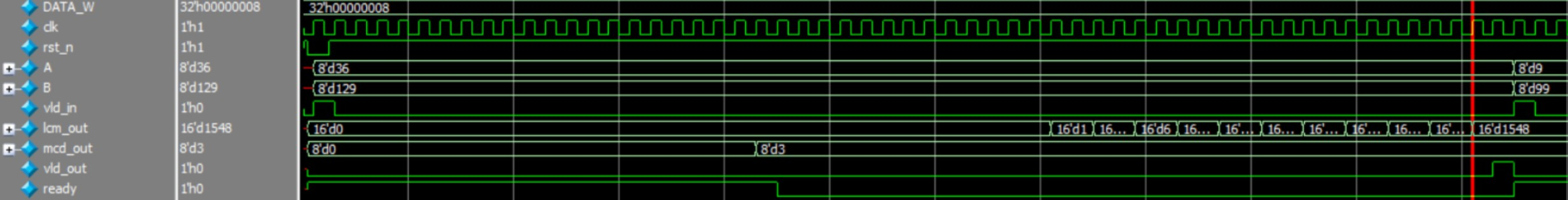``````//------------------------------------------------------------------------------
//
//Module Name:					LCM.v
//Department:					Xidian University
//Function Description:	        求最大公约数和最小公倍数
//
//------------------------------------------------------------------------------
//
//Version 	Design		Coding		Simulata	  Review		Rel data
//V1.0		Verdvana	Verdvana	Verdvana		  			2020-01-04
//
//-----------------------------------------------------------------------------------
//
//Version	Modified History
//V1.0
//
//-----------------------------------------------------------------------------------

`timescale 1ns/1ns

module LCM #(
parameter   DATA_WIDTH = 8          //数据位宽
)(
//**********时钟&复位***********//
input                       clk,    //时钟
input                       rst_n,  //复位
//**********控制信号***********//
input                       en,     //使能
//**********输入数据***********//
input  [DATA_WIDTH-1:0]     a,      //第一个数
input  [DATA_WIDTH-1:0]     b,      //第二个数
//**********输出数据***********//
output                      valid,  //输出数据有效信号
output [DATA_WIDTH*2-1:0]   gcd,    //最大公约数
output [DATA_WIDTH*2-1:0]   lcm     //最小公倍数
);

//===================================================
//使能上升沿检测

reg en_r1,en_r2;

always_ff @(posedge clk, negedge rst_n) begin
if(!rst_n) begin
en_r1 <= '0;
en_r2 <= '0;
end
else begin
en_r1 <= en;
en_r2 <= en_r1;
end
end

wire enable;

assign enable = en_r1 && ~en_r2;

//===================================================
//辗转相除计算最大公约数

reg  [DATA_WIDTH-1:0] numer,denom;

wire [DATA_WIDTH-1:0]quotient,remain;

DIV#(
.DATA_WIDTH(DATA_WIDTH)                     //数据位宽
)u_DIV(
.numer,      //被除数
.denom,      //除数

.quotient,   //商
.remain      //余数
);

reg [DATA_WIDTH*2-1:0]   gcd_r;

always_ff @(posedge clk, negedge rst_n) begin
if(!rst_n) begin
numer <= '0;
denom <= '0;
end
else if(valid) begin
numer <= '0;
denom <= '0;
end
else if(enable) begin
numer <= a;
denom <= b;
end
else if(remain!='0)begin
numer <= denom;
denom <= remain;
end
else begin
gcd_r <= denom;
end
end

assign gcd = (valid)?gcd_r:'0;

//===================================================
//产生输出有效信号

reg valid_r;

always_ff @(posedge clk, negedge rst_n) begin
if(!rst_n) begin
valid_r <= '0;
end
else if((remain=='0)&&(denom!='0)&&(valid_r=='0)) begin
valid_r <= '1;
end
else begin
valid_r <= '0;
end
end

assign valid = valid_r;

//===================================================
//计算最小公倍数

wire [DATA_WIDTH*2-1:0]   lcm_r;
wire [DATA_WIDTH*2-1:0]   product;

assign product = a*b;

DIV#(
.DATA_WIDTH(DATA_WIDTH*2)                     //数据位宽
)ulcm_DIV(
.numer(product),      //被除数
.denom(gcd),      //除数

.quotient(lcm_r),   //商
.remain()      //余数
);

assign lcm = (valid)?lcm_r:'0;

endmodule
``````
``````//------------------------------------------------------------------------------
//
//Module Name:					DIV.v
//Department:					Xidian University
//Function Description:	        整数除法器
//
//------------------------------------------------------------------------------
//
//Version 	Design		Coding		Simulata	  Review		Rel data
//V1.0		Verdvana	Verdvana	Verdvana		  			2020-01-03
//
//-----------------------------------------------------------------------------------
//
//Version	Modified History
//V1.0
//
//-----------------------------------------------------------------------------------

module DIV #(
DATA_WIDTH  = 8                     //数据位宽
)(
//**********输入***********//
input  [DATA_WIDTH-1:0] numer,      //被除数
input  [DATA_WIDTH-1:0] denom,      //除数
//**********输出***********//
output [DATA_WIDTH-1:0] quotient,   //商
output [DATA_WIDTH-1:0] remain      //余数
);

reg [DATA_WIDTH*2-1:0] numer_r;
reg [DATA_WIDTH*2-1:0] denom_r;

reg quotient_e [DATA_WIDTH];

always_comb begin
numer_r = ,numer};   //左边补齐0
denom_r = denom << DATA_WIDTH;          //右边补齐0
end

reg [DATA_WIDTH*2-1:0] numer_e [DATA_WIDTH+1];
reg [DATA_WIDTH*2-1:0] denom_e [DATA_WIDTH+1];

assign numer_e = numer_r;
assign denom_e = denom_r >> 1;

generate
genvar i;
for(i=0;i<DATA_WIDTH;i++)
begin:shift
always_comb begin
if( numer_e[i] >= denom_e[i])begin
quotient_e[DATA_WIDTH-1-i] = 1'b1;
numer_e[i+1] =  numer_e[i]-denom_e[i];
denom_e[i+1] = denom_e[i] >> 1;
end
else begin
quotient_e[DATA_WIDTH-1-i] = 1'b0;
numer_e[i+1] =numer_e[i];
denom_e[i+1] = denom_e[i] >> 1;
end

end

assign quotient[i] = quotient_e[i]; //得出商
end
endgenerate

assign remain = numer_e[DATA_WIDTH];    //取余数

endmodule
``````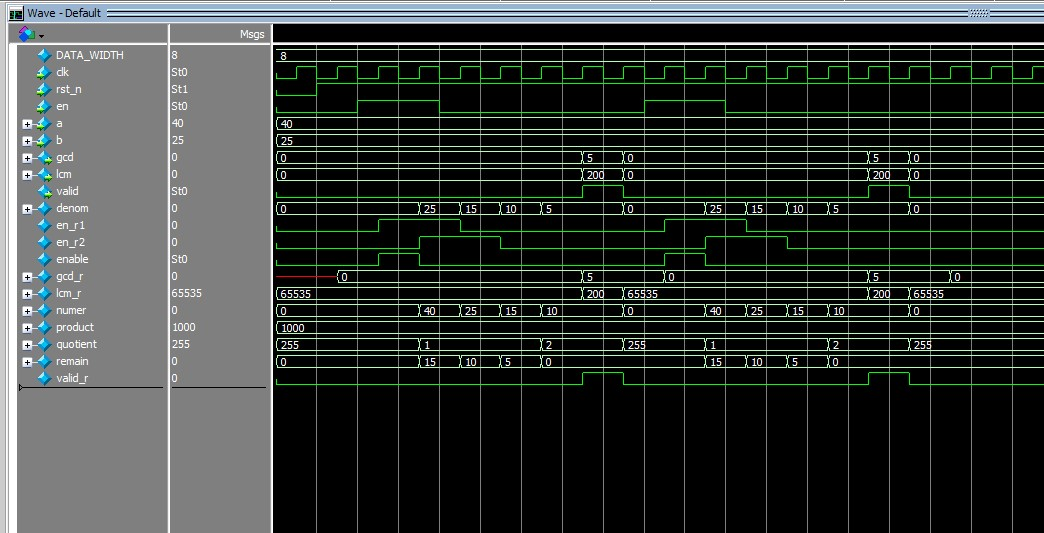告辞。